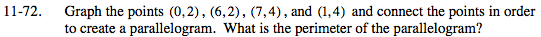### Home > MC2 > Chapter 11 > Lesson 11.2.4 > Problem11-72

11-72.In order to find the perimeter, we need to know the lengths of all 4 sides of the parallelogram. We know two of the sides from the graph. How can we find the lengths of the other two sides?

Draw a triangle so that the missing side is the hypotenuse of the triangle. Then use the Pythagorean Theorem to find the length of the hypotenuse.

1² + 2² = hypotenuse²
1 + 4 = hypotenuse²
5 = hypotenuse²

$\text{hypotenuse }=\; \sqrt{5}$

Now add all the sides together to get the perimeter of the parallelogram.

Does the answer repeat or terminate?

Use the eTool below to graph the points and create a parallelogram.
Click the link at right for the full version of the eTool: MC2 11-72 HW eTool